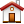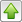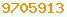# BigDecimal : Java Glossary

BigDecimal
java.math.BigDecimal provides for immutable arbitrary-precision signed decimal numbers. A BigDecimal consists of an arbitrary precision integer unscaled value (a BigInteger 2’s complement variable length array of bytes) and a non-negative 32-bit integer scale, which represents the number of digits to the right of the decimal point. Scales may not be negative. That allows you to work with decimal fractions of arbitrary precision.

To convert a long to a BigDecimal use:

```// convert a long to BigDecimal, with some implied decimal places.
BigDecimal d = BigDecimal.valueOf( theLong, decimalPlaces );```

You can’t use ordinary arithmetic or comparison operators on BigDecimal. You must use methods like  BigDecimal.add, .subtract, .multiply,. divide, .pow, .abs,.compareTo

To convert your BigDecimal to a String for display you can use BigDecimal. toString or BigDecimal. toEngineeringString. It is also possible using Formatter and printf.

Watch out! ==, BigDecimal.equals and BigDecimal.compareTo() == 0 all give different results.
• == returns true only if the two BigDecimal references to the same object. It is not sufficient they have the same value.
• Unlike compareTo, equals considers two BigDecimal objects equal only if they are equal in value and scale (thus 2.0 is not equal to 2.00 when compared by this method).
• Two BigDecimal objects that are equal in value but have a different scale (like 2.0 and 2.00) are considered equal by compareTo.

PSPDec (Prospero Software Products Decimal) is a third party library.

## Learning More

Oracle’s Javadoc on BigDecimal class : available:
Arcimath
BigInteger
DecimalD Spec
gotchas
IBM’s BigDecimal: a cleanroom implementation of BigDecimal
PSPDecThis page is postedon the web at: http://mindprod.com/jgloss/bigdecimal.html Optional Replicator mirror of mindprod.com on local hard disk J: J:\mindprod\jgloss\bigdecimal.htmlPlease read the feedback from other visitors, or send your own feedback about the site.Contact Roedy. Please feel free to link to this page without explicit permission. Canadian Mind Products IP:[65.110.21.43] Your face IP:[54.243.17.113] Feedback You are visitor number